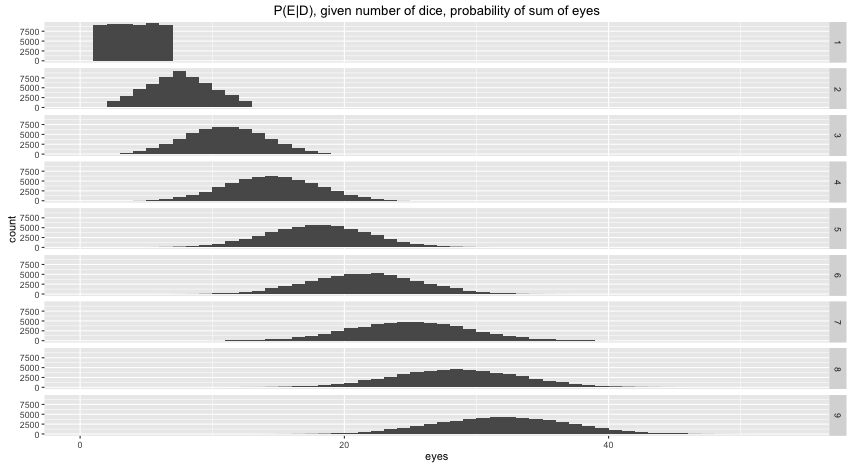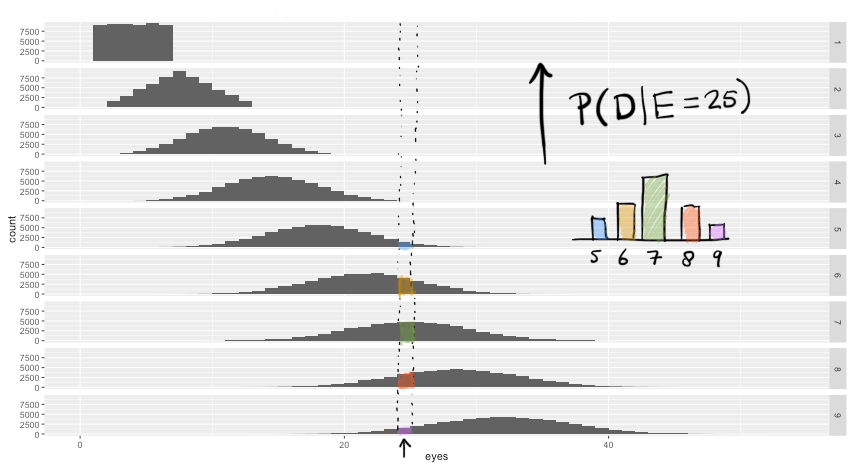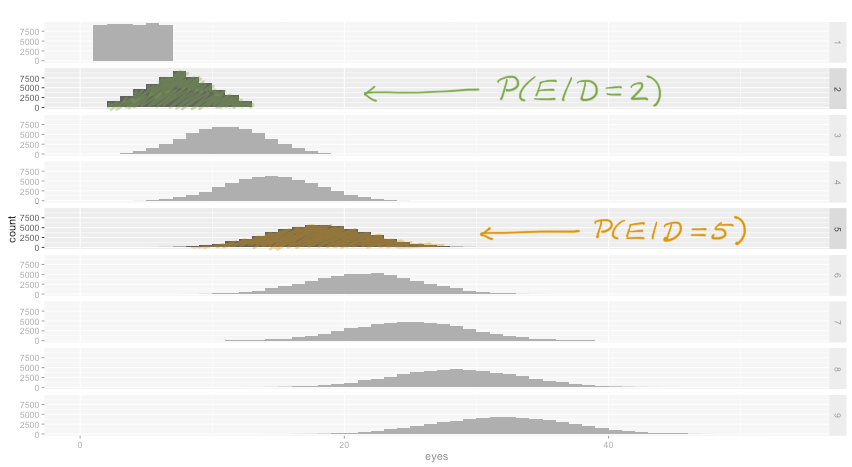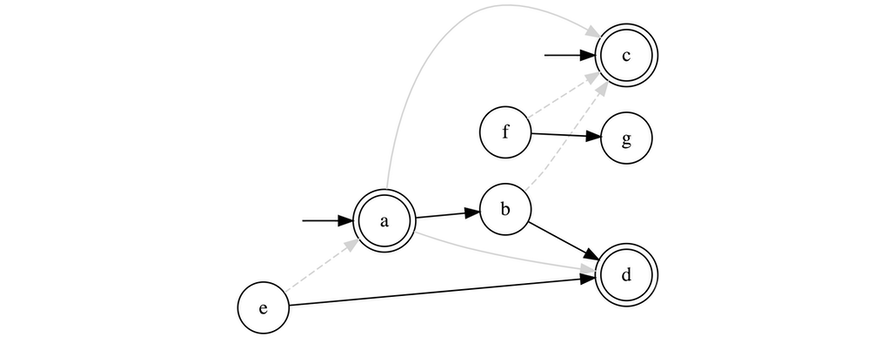# Introduction to Inference

Reduce Common Sense to Calculus.

Vincent Warmerdam koaning.io
02-15-2020

“The theory of probabilities is just common sense reduced to calculus.”

– Pierre-Simon Laplace

This document contains an introduction to probibalistic inference meant as a comfortable first step for data people to get introduced to bayesian thinking. It will be a bit mathy, but nothing beyond kahn-level probability. It is a repost of an old document. I will assume you’ve done textbook exercises in probability theory before and that you’ve heard of bayes rule as well.

# Definition

Bayes Rule is a lovely law in probability theory that is defined via a simple formula.

$P(E|D) = \frac{P(E,D)}{P(D)} = \frac{P(D|E)P(E)}{P(D)}$

Here $$E$$ and $$D$$ are events. They can be anything. As a simplest example; suppose that I am rolling dice and that $$D$$ is the number of dice that I’ve rolled and that $$E$$ is the number of eyes that I see. Via simulation of dice I can generate plots of what $$P(E|D)$$ might look like.Figure 1: When we roll more dice, the expect a different number of eyes.

In this plot you see the probability distribution for the number of eyes given the number of dice that are rolled. The number of dice are listed on the right hand side, the number of eyes are denoted on the x-axis and the y-axis shows how often this occured in a simulation. You should notice that when I roll 4 dice that it is not likely for me witness a total of 4 eyes but it is impossible to get 3. This is what we expect and this is what we could also calculate with probability theory.

Here’s an amazing thing though; you could turn this plot on it’s head. If you only switch the numbers on the x-axis with the numbers on the right hand side then you suddenly have all distributions for $$P(D|E)$$. We can use what we know about $$P(E|D)$$ to gain knowledge about $$P(D|E)$$. As we’ll see in the next formal example, this is a very nice property and it’s something that follows straight from probability theory.Figure 2: Same simulated data, but two different questions you can ask it. Here P(D|E)Figure 3: Same simulated data, but two different questions you can ask it. Here P(D|E)

It’s not just pretty colors. There’s many benefits of this way of thinking.

# Textbook Example

Let’s discuss a textbook example, one that I remember from my exams.

There is an epidemic. A person has a probability 1/100 to have the disease. The authorities decide to test the population, but the test is not completely reliable: the test generally gives 1/110 people a positive result but given that you have the disease the probability of getting a positive result is 65/100.

Let $$D$$ denote the event of having the disease, let $$T$$ denote event of a positive outcome of a test. If we are interested in finding $$P(D|T)$$ then we can just go and apply Bayes rule:

$P(D|T) = \frac{P(T|D)P(D)}{P(T)} = \frac{\frac{65}{100} \times \frac{1}{100}}{\frac{1}{110}} \approx 0.715$

This is neat. We learned something about having the disease by knowing somthing on the performance of the test. Bayes rule gives us a precise method to generate more knowledge after having observed data. In this case the data that we observe is the fact that the test was positive. What else can we calculate?

# Infer Moar!

Suppose that you do not have the disease, can the test come out positive? In maths: what is $$P(T| \neg D)$$?

We can use basic probability theory again and split up the probability of getting a positive test result. It’s easier to think about this outcome by splitting it up into two situations; the situation that multiple tests are positive given that you have the disease and the situation that multiple tests are positive given that you don’t have the disease.

\begin{align} P(T) & = P(T\cap D) + P(T\cap \neg D) \\ & = P(T|D)P(D) + P(T|\neg D)P(\neg D) \end{align}

We know $$P(T|D)$$ , $$P(D)$$ and $$P(T)$$. We can figure out that $$P(¬D)=1−P(D)$$ too. So we only need to fill these numbers into the formula above to get;

$P(T|\neg D) = \frac{19}{7260}$

# Infer Even Moar!

Sofar, we’ve only considered the outcome of one test. But a person might seek a second opinion and go to another doctor. If we assume that these two doctors don’t influence eachother then again we can think about what probability theory has to say about this. So what are the probabilities from two or three tests being positive?

\begin{align} P(TT) &= P(TT|D)P(D) + P(TT| \neg D) P(\neg D) &\approx 0.004237374 \\ P(TTT) &= P(TTT|D)P(D) + P(TTT| \neg D) P(\neg D) &\approx 0.002746294 \end{align}

Derivation of the Maths. You might be wondering how $$P(TT|D)$$ and $$P(TT | \neg D)$$ are calculated. The main trick here is realising that $$P(TT | D) = P(T | D) \times P(T | D)$$.

So why does that make sense? If you take two tests, surely they are related, no?

This is true, but only if we don’t know if you have the disease. If we know that you have the disease then the doctors you will see might disagree but in general they should tell you 65% of the time that you have the disease. It is assumed that the doctors don’t influence eachother. This means that each test result that each doctor gives you must be independant.

\begin{align} P(TT) &= P(TT|D)P(D) + P(TT| \neg D) P(\neg D) \\ &= P(T|D) P(T|D)P(D) + P(T| \neg D) P(T| \neg D) P(\neg D) \\ &= P(T|D)^2 P(D) + P(T| \neg D)^2 P(\neg D) \end{align} This also holds for more tests.

\begin{align} P(TTT) &= P(TTT|D)P(D) + P(TTT| \neg D) P(\neg D) \\ &= P(T|D)^3 P(D) + P(T| \neg D)^3 P(\neg D) \end{align} You might still be confused. Why $$P(TT) \neq P(T) \times P(T)$$ while $$P(TT | D) = P(T|D) \times P(T|D)$$?

Understanding this properly takes a while (my experience). For now I hope you reliase that $$P(TT|D)$$ can be interpreted as a query. It asks for the likelihood of seeing two positive tests, given that you have the disease. $$P(TT)$$ is a strictly different query.

The next part of the post contains diagrams that might also help to understand this.

Notice that these probabilities were not strictly given to us. These are probabilities that we’ve inferred from the information known to us by applying probability theory. The only true assumption that we’ve made is that the only thing that influeces a test result from a doctor is the presence of the disease (i.e. the doctors are not allowed to influence eachother beyond that).

# Graphical Views

Let’s make a drawing of the causual graph next to our original formula.

\begin{align} P(D|T) & = \frac{P(T|D)P(D)}{P(T)} = \frac{\frac{65}{100} \times \frac{1}{100}}{\frac{1}{110}} \\ & \approx 0.715 \end{align}

The graph says that the disease is what has an effect on the test result. Not the other way around. This makes sense. It’s not the test that makes you sick. The fact that you are sick makes the test result more likely to be positive. It is this information that the causal chart demonstrates. Nothing about probability values, just the causal relationships.

## Two Tests

Let’s move on to calculate something else that is interesting; what is the probability of having the disease after two doctors do two tests on you? We’ll write down the maths and draw the causal diagram again.

\begin{align} P(D|TT) & = \frac{P(TT|D)P(D)}{P(T)} \\ & \approx 0.9971 \end{align}

Note that we are now more certain! After seeing two positive test results it is harder to assume you don’t have the disease.

How to calculate $$P(TT|D)$$. The Rule-Of-Total-Probability[tm] states that;

\begin{align} P(T) & = P(T\cap D) + P(T\cap \neg D) \\ & = P(T|D)P(D) + P(T|\neg D)P(\neg D) \end{align} In words; there’s only two options if you have a positive test. It is positive and you’ve got the disease or it’s positive and you don’t. By splitting this probability into two seperate quantities to calculate we get a formula with 5 items. Out of these we know $$P(T)$$, $$P(D)$$ and $$P(\neg D)$$. What about $$P(T|D)$$ and $$P(T|\neg D)$$?

$$P(T|D)$$ should not be confused with $$P(D|T)$$, but we can use bayes rule to use $$P(D|T)$$ to calculate $$P(T|D)$$.

$P(T|D) = \frac{P(D , T)}{P(D)} = \frac{P(D|T)P(T)}{P(D)}$ That formula contains $$P(D|T), P(T)$$ and $$P(D)$$. These are all known. A similar conclusion can be made for the situation with $$\neg D$$

$P(T| \neg D) = \frac{P(\neg D , T)}{P(\neg D)} = \frac{P(\neg D|T)P(T)}{P(\neg D)}$ Because $$P(\neg D) = 1 - P(D)$$ and $$P(\neg D | T) = 1 - P(D | T)$$ we again have everything we need.

To calculate $$P(TT|D)$$ we merely need to multiply since the two tests are independant $$P(TT|D) = P(T|D) \times P(T|D)$$.

## Three Tests

Let’s move on to calculate something else that is interesting; what is the probability of having the disease after two doctors do two tests on you? We’ll write down the maths and draw the causal diagram again.

\begin{align} P(D|TTT) & = \frac{P(TTT|D)P(D)}{P(T)} \\ & \approx 0.9999 \end{align}

This results makes sense. After seeing three doctors, it starts getting pretty likely that you actually have the disease. We also see that the added certainty of doing a third test after seeing two positives is low. We gain little information.

# Pretty Amazing

With the help of Bayes’ Rule we’ve just inferred information about $$P(D | TTT)$$ even though we initially only started with $$P(T|D)$$, $$P(T)$$ and $$P(D)$$.

Part of the trick here lies in the causal graph. It defines a model of the world. By assuming that the disease is the only thing that can influence a test outcome we can infer the rest. But it is exactly this makes inference so strong! By defining the causal direction between variables (common sense) we can calculate the rest (calculus).

# Even Moar?

Let’s draw the diagram again but now differently. We’ll now put in a question-mark when we don’t know the variable. That way we can encode a query into a pretty picture. So let’s draw one for $$P(T_3 | T_1, T_2)$$. In words this means “Given that you’ve done two tests that are both positive, how likely is it that the third one is also positive?”

The maths for this query is listed below.

$P(T_3 | T_1 T_2 ) = \frac{P(T_1, T_2, T_3)}{P(T_1, T_2)} = \frac{1076657}{1661220} \approx 0.6481$

Alternative Derivation. The above derivation works, but there is an alternative.

\begin{align} P(T_3 | T_1, T_2) & = P(T_3 | T_1, T_2, D) P(D | T_1, T_2) + P(T_3 | T_1, T_2, \neg D) P(\neg D | T_1, T_2) \\ & = P(T_3 | D) P(D | T_1, T_2) + P(T_3 | \neg D) P( \neg D | T_1, T_2) \\ &\approx 0.6481 \end{align} To get here it helps to realise that;

\begin{align} P(D | T_1, T_2) & = \frac{16731}{16780} \\ P(\neg D | T_1, T_2) & = 1 - P(D | T_1, T_2) = \frac{49}{16780} \end{align} I hope you can infer the benefits of the former method over the latter.

You can describe the sensation of inference through words as well. If two tests are positive, it is very likely that you have the disease. If it is very likely that you have the disease then it is also rather likely that the next test is positive. If you look at the graph, the inference that we do on it has a certain direction. We infer knowledge of $$T_3$$ via $$D$$.

The graphical representation tells us that once we know $$D$$ the events $$T1...T3$$ are independant of eachother. That doesn’t mean that we cannot infer about $$P(D|T_i)$$, it merely means that we have to keep track of different assumptions in our model. Probability theory resolves the rest.

# Conclusion

Please recognize how powerful this methodology is. Not only does this way of thinking give you immense modelling flexibilities but it even allows you to work with actual probabilities at all times. Many machine learning models try to approximate this thinking with more black box sort of methods but it loses some probibalistic interpretation in the process.

## Important Detail

In this blogpost I’ve discussed a simple example that demonstrates the elegant parts of Bayes’ rule. But the model on display here might not be the best way of thinking about the world. The assumption that tests do not influence eachother is a big one. If you see a second doctor from the same hospital then odds are that this doctor will not have a completely independant opinion.

To accomodate for this you might need another way of looking at the world. A more hierarchical way of looking at it. So we might need to update our causal graph.

Maybe a causal model like this;Figure 4: May be a better model. D is for disease, T is for a test/doctor, H is for hospital

This graph tells us qualitatively how variables are related but now we’ve gotta come up worth datasets that help us to quantify the effects between variables.

No free lunch here; modelling is still very hard.

# Experiment

I have an alpha (ALPHA!) open source project that tries to make these calculations easy. It is unlikely that I’ll be able to offer any support for it anytime soon but it might be fun to play with.

It gives you an overview of both causaul graphs as well as plots of the queries you send it. Feed it a dataframe with discrete variables and you can ask it questions.Figure 5: Good lookin’ graphics!

They’re good DAGS and the project is called brent. Documentation found here.

# Blog Tech Revamp

I was really exited to redo this blogpost because it allowed me to demonstrate a really cool html5 rick. Notice those blocks you can click that will expand? That’s all html, no javascript.


<details>
<summary><b>Alternative Derivation.</b></summary>
Blah blah text and explain, maybe an image?
</details>

This is amazing. I often wonder if I should include details in the blogpost and now I can leave it to the end-user to explore details if needed. This saves on screen real-estate and also makes the post a lot less intimidating to explore.

The medium for the content should invite people, not scare them away. Maths unfortunatly is a language that is great for precision of details but bad for recall of inclusion.